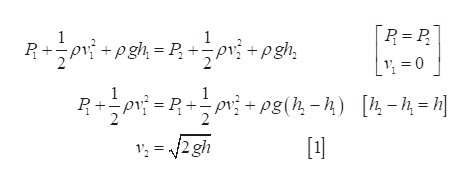# A large storage tank, open to the atmosphere at the top and filled with water, develops a small hole in its side at a point 16.0 m below the water level. If the rate of flow from the leak is 2.50 x 10-3 m3 /min, determine (a) the speed at which the water leaves the hole and (b) the diameter of the hole.

Question
28 views

A large storage tank, open to the atmosphere at the top and filled with water, develops a small hole in its side at a point 16.0 m below the water level. If the rate of flow from the leak is 2.50 x 10-3 m3 /min, determine (a) the speed at which the water leaves the hole and (b) the diameter of the hole.

check_circle

Step 1

(a) Using the Bernoulli’s equation at the top and the hole.help_outlineImage Transcriptionclose[R = P, R+pr; + pgh = P, +pv + pgh, R+pr =R+pr - pg(4, – 4) pv + pg(h, - h) [½-4 = h] [4 - = ||  1; = /2 gh fullscreen
Step 2

Substitute the value in equation (1) to find the speed at which the water leaves the hole.

Step 3

(b) The rate of...

### Want to see the full answer?

See Solution

#### Want to see this answer and more?

Solutions are written by subject experts who are available 24/7. Questions are typically answered within 1 hour.*

See Solution
*Response times may vary by subject and question.
Tagged in

### Other